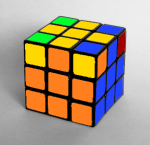# Solving The Final 4 Corners of the Rubik's CubeIn the penultimate lesson on the Rubik's Cube, the final four corners are positioned using one algorithm and solved using the repetition of another. This in turn solves the cube and completes the solving section of this series. A similar algorithm is used to rotate the corners as was used in the first four corner solution.

## Recap and Overview

As always, the lesson is begun with a recap of the previous lesson's content. Here the student is tested on some concepts and algorithms that they have learnt in each different lesson, and encourages them to explain the purpose of algorithms they provide. The answers are then provided on the following slide.

Following the standard recap questions, the student is introduced to the two parts of the stage that they will be completing in this lesson. An overview of the lesson content is covered, followed by another notice that they need to be able to solve the cube up to this point to be able to learn the following parts properly.

## Positioning the final 4 corners

The first of the two parts of the solve will correctlyposition the 4 corners so that they only need to be rotated to solve the cube, whilst making sure that what has already been solved (the rest of the cube) isn't disturbed.

An algorithm that cycles three of the four top corners is given. This will be used to correctly position all four corners. Most of the time, the student will have at least one corner already in its correct position, however this isn't a necessity. The student is told to preserve the corner that is already solved (if there is one) by holding the cube so that the piece they want to preserve is in the position that isn't affected by the algorithm that they are about to perform.

## Rotating the final 4 corners

The second of the two parts, therotation of the final 4 corners uses an algorithm that needs to be repeated in the same way that the algorithm they learnt to solve the first four corners of the cube needed to be repeated. The student is taught how to identify the number of times that they will have to perform the algorithm by looking at the permutation of the corner in question.

Once they have figured out the number of times that they have to perform the algorithm, they are taught how to perform the algorithm several times on different corners whilst keeping the rest of the solved pieces intact. Animations are provided on the following slide to help students better understand what they need to do.

Finally, the student is congratulated for completing the Rubik's Cube. If they are unable to complete the cube, then they need to return to previous presentations to relearn the stages.

#### Rubik's Cube Curriculum SeriesDownload The PowerPoint Presentations from Google Drive